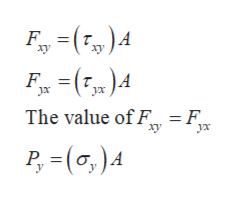# А.300В

Question

Determine the stress components acting on the inclined plane AB in if:

normal stressy = 61MPa

shear stressxy = -25 MPa

solve the problem using the method of equilibrium.

Find normal stress x' =?

find shear stress x'y' =?

check_circle

Step 1

Consider the following diagram representing stresses acting on the body. The area of cross-section of the body is A.

Step 2

The body is subjected to uniaxial tensile and shear stress (i.e. acting in the plane x-y) as shown in the above diagram. Consider free body diagram of the section OAB.

Step 3

Therefore, the magnitude o...help_outlineImage TranscriptioncloseF ху -(Fm)4 F Jх = F_ The value of F xy ух P -(0,)A Р, fullscreen

### Want to see the full answer?

See Solution

#### Want to see this answer and more?

Solutions are written by subject experts who are available 24/7. Questions are typically answered within 1 hour.*

See Solution
*Response times may vary by subject and question.
Tagged in

### Mechanical Engineering